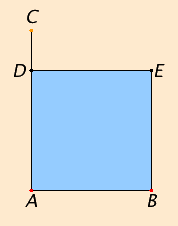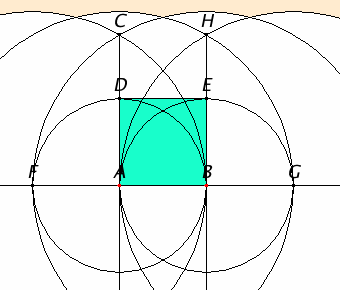# Proposition 46

To describe a square on a given straight line.

Let AB be the given straight line.

It is required to describe a square on the straight line AB.Draw AC at right angles to the straight line AB from the point A on it. Make AD equal to AB. Draw DE through the point D parallel to AB, and draw BE through the point B parallel to AD.

I.34

Then ADEB is a parallelogram. Therefore AB equals DE, and AD equals BE.

But AB equals AD, therefore the four straight lines BA, AD, DE, and EB equal one another. Therefore the parallelogram ADEB is equilateral.

I say next that it is also right-angled.

I.29

Since the straight line AD falls upon the parallels AB and DE, therefore the sum of the angles BAD and ADE equals two right angles.

I.Post.4

But the angle BAD is right, therefore the angle ADE is also right.

I.34

And in parallelogrammic areas the opposite sides and angles equal one another, therefore each of the opposite angles ABE and BED is also right. Therefore ADEB is right-angled.

And it was also proved equilateral.

I.Def.22

Therefore it is a square, and it is described on the straight line AB.

Q.E.F.

## Guide

We now have the second regular polygon, the first being the equilateral triangle of proposition I.1. Regular polygons with 5, 6, and 15 sides are constructed in Book IV.

#### Construction steps

 There are quite a few steps needed to construct a square on AB. In order to construct the perpendicular AC, first AB has to be extended in the direction of A and a point F on the far side the same distance from A as B is, then two more circles centered at B and F to get a perpendicular line, and then it needs to be cut off at length C, but fortunately, the needed circle has already been drawn. Next, EB is to be drawn through B parallel to AD. In general that construction given in I.31 takes six circles, but in this case if EB is drawn perpendicular to AB, then it will be parallel to AD, too, and that construction only takes three circles with radii BA, AG, and GA. This abbreviation of Euclid’s construction requires six circles and four lines. There are alternate constructions that are a bit shorter.#### Use of Proposition 46

The construction of a square given in this proposition is used in the next proposition, numerous propositions in Book II, and others in Books VI, XII, and XIII.